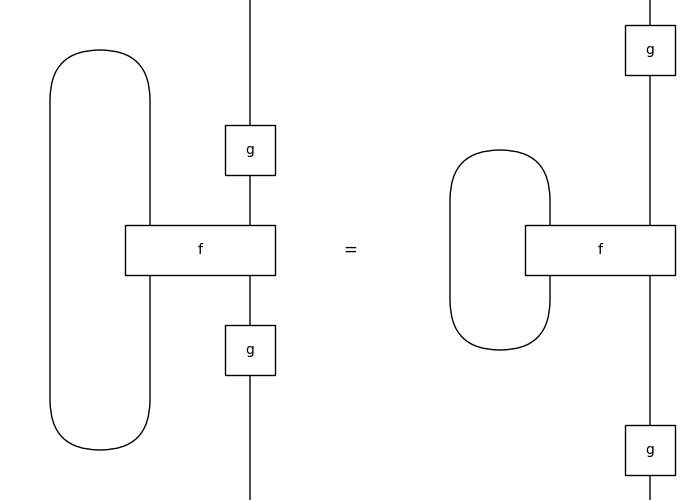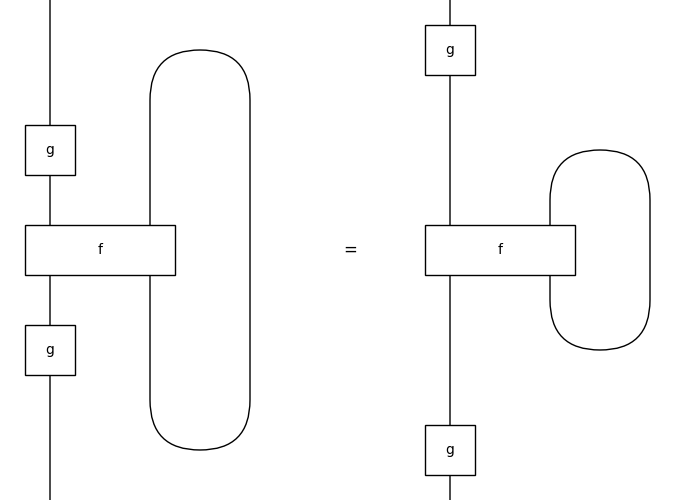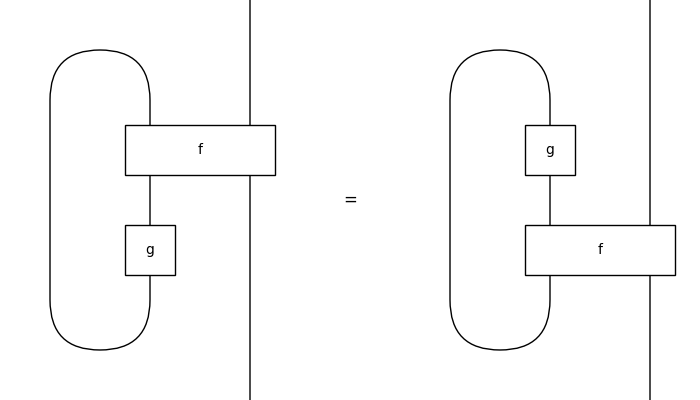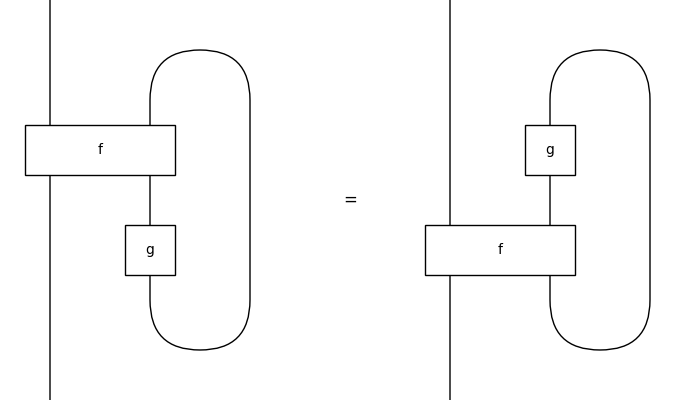# traced#

The free traced category, i.e. diagrams where outputs can feedback into inputs.

Note that these diagrams are planar traced so that e.g. `pivotal` diagrams are traced in this sense. See `symmetric` for the usual notion of trace.

Whenever the diagrams are also `symmetric`, their equality can be checked by translation to monogamous `hypergraph`.

## Summary#

 `Diagram` A traced diagram is a monoidal diagram with `Trace` boxes. `Box` A traced box is a monoidal box in a traced diagram. `Trace` A trace is a diagram `arg` with an output wire fed back into an input. `Category` A traced category is a monoidal category with a method `trace`. `Functor` A traced functor is a monoidal functor that preserves traces.

## Axioms#

```>>> from discopy.drawing import Equation
>>> from discopy.symmetric import Ty, Box, Swap, Id
>>> from discopy import symmetric
>>> symmetric.Diagram.structure_preserving = True
>>> x = Ty('x')
>>> f, g = Box('f', x @ x, x @ x), Box('g', x, x)
```
• Vanishing

```>>> assert f.trace(n=0) == f == f.trace(n=0, left=True)
>>> assert f.trace(n=2) == f.trace().trace()
>>> assert f.trace(n=2, left=True) == f.trace(left=True).trace(left=True)
```
• Superposing

```>>> assert (x @ f).trace() == x @ f.trace()
>>> assert (f @ x).trace(left=True) == f.trace(left=True) @ x
```
• Yanking

```>>> yanking = Equation(
...     Swap(x, x).trace(left=True), Id(x), Swap(x, x).trace())
>>> yanking.draw(
...     path='docs/_static/traced/yanking.png', draw_type_labels=False)
``````>>> assert yanking
```
• Naturality

```>>> tightening_left = Equation(
...     (x @ g >> f >> x @ g).trace(left=True),
...     g >> f.trace(left=True) >> g)
>>> tightening_left.draw(
...     path='docs/_static/traced/tightening-left.png', draw_type_labels=False)
``````>>> tightening_right = Equation(
...     (g @ x >> f >> g @ x).trace(),
...     g >> f.trace() >> g)
>>> tightening_right.draw(
...     path='docs/_static/traced/tightening-right.png',
...     draw_type_labels=False)
``````>>> assert tightening_left and tightening_right
```
• Dinaturality

```>>> sliding_left = Equation(
...     (f >> g @ x).trace(left=True),
...     (g @ x >> f).trace(left=True))
>>> sliding_left.draw(
...     path='docs/_static/traced/sliding-left.png', draw_type_labels=False)
``````>>> sliding_right = Equation(
...     (f >> x @ g).trace(),
...     (x @ g >> f).trace())
>>> sliding_right.draw(
...     path='docs/_static/traced/sliding-right.png', draw_type_labels=False)
``````>>> assert sliding_left and sliding_right
```
```>>> symmetric.Diagram.structure_preserving = False
```